• BP神经网络：误差反向传播算法公式推导 开端： BP算法提出 1. BP神经网络参数符号及激活函数说明 2. 网络输出误差(损失函数)定义 3. 隐藏层与输出层间的权重更新公式推导 4. 输入层与隐藏层间的权重更新...

BP神经网络：误差反向传播算法公式推导

1. BP神经网络参数符号及激活函数说明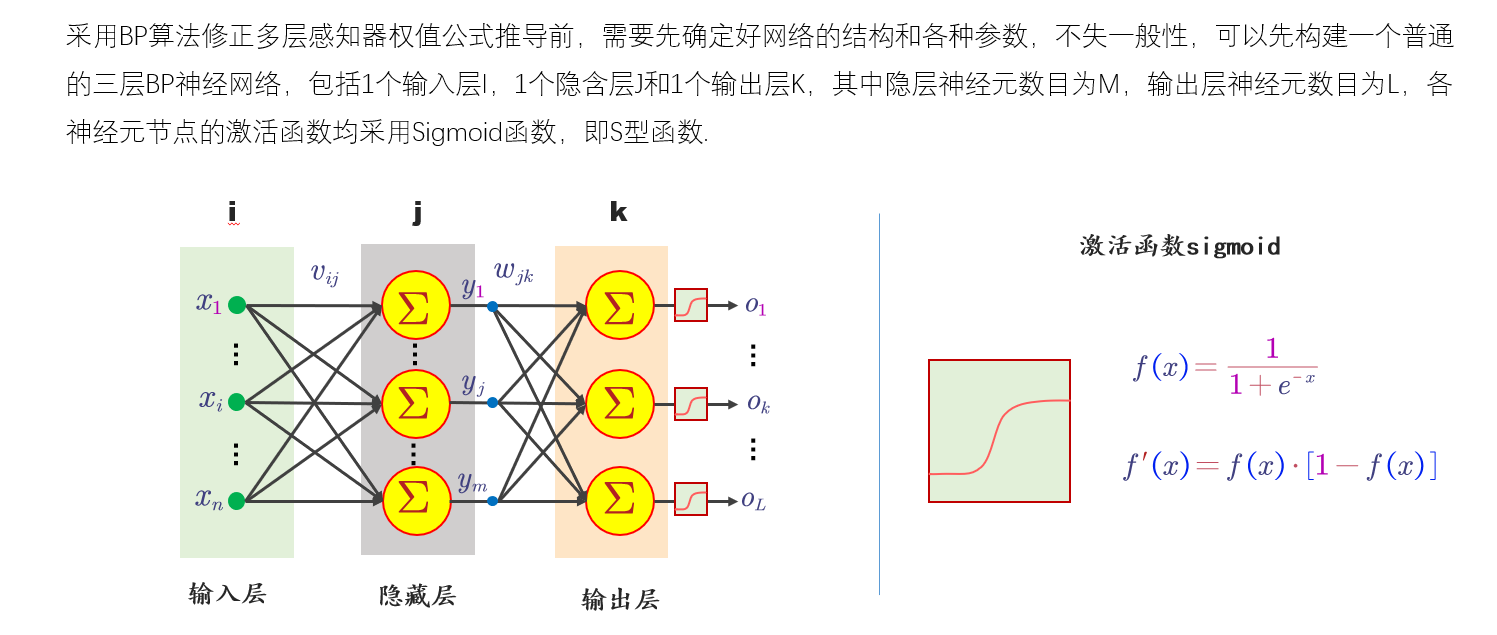3. 隐藏层与输出层间的权重更新公式推导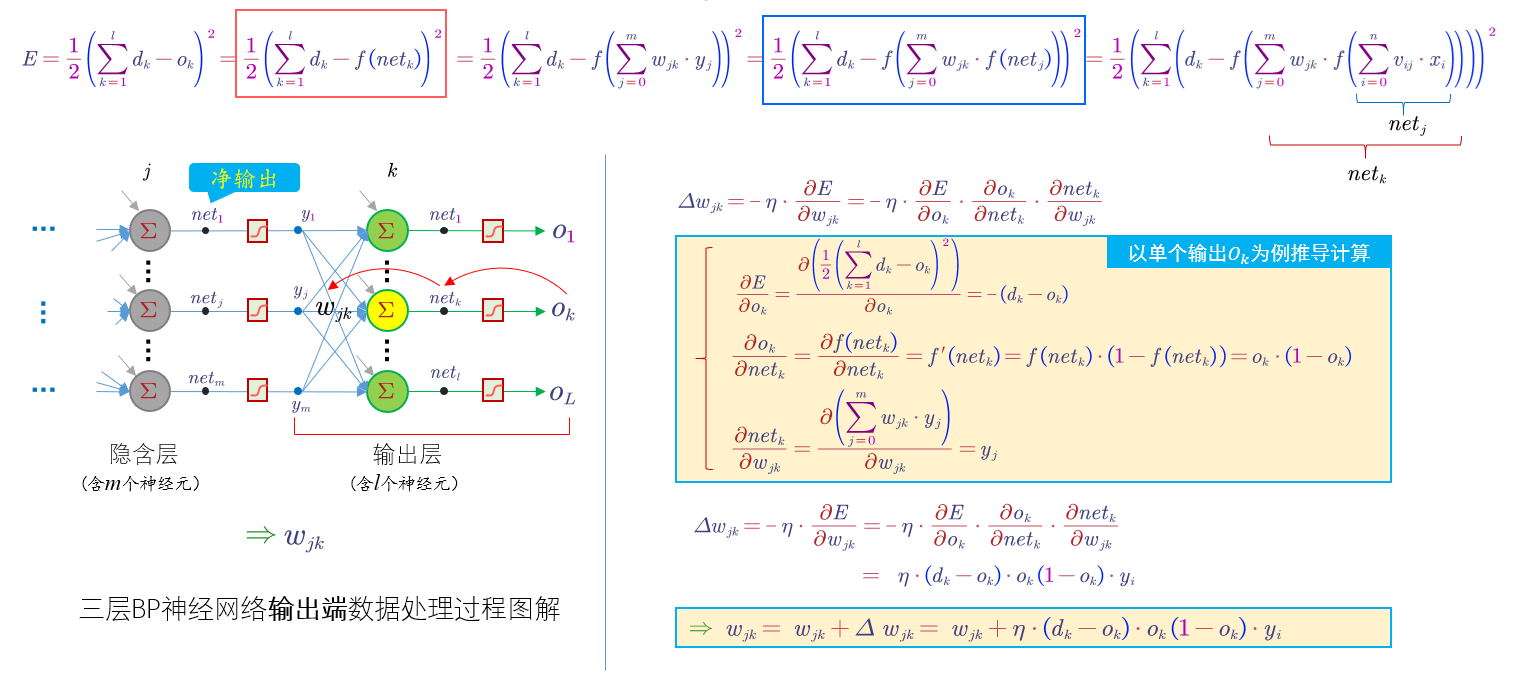4. 输入层与隐藏层间的权重更新公式推导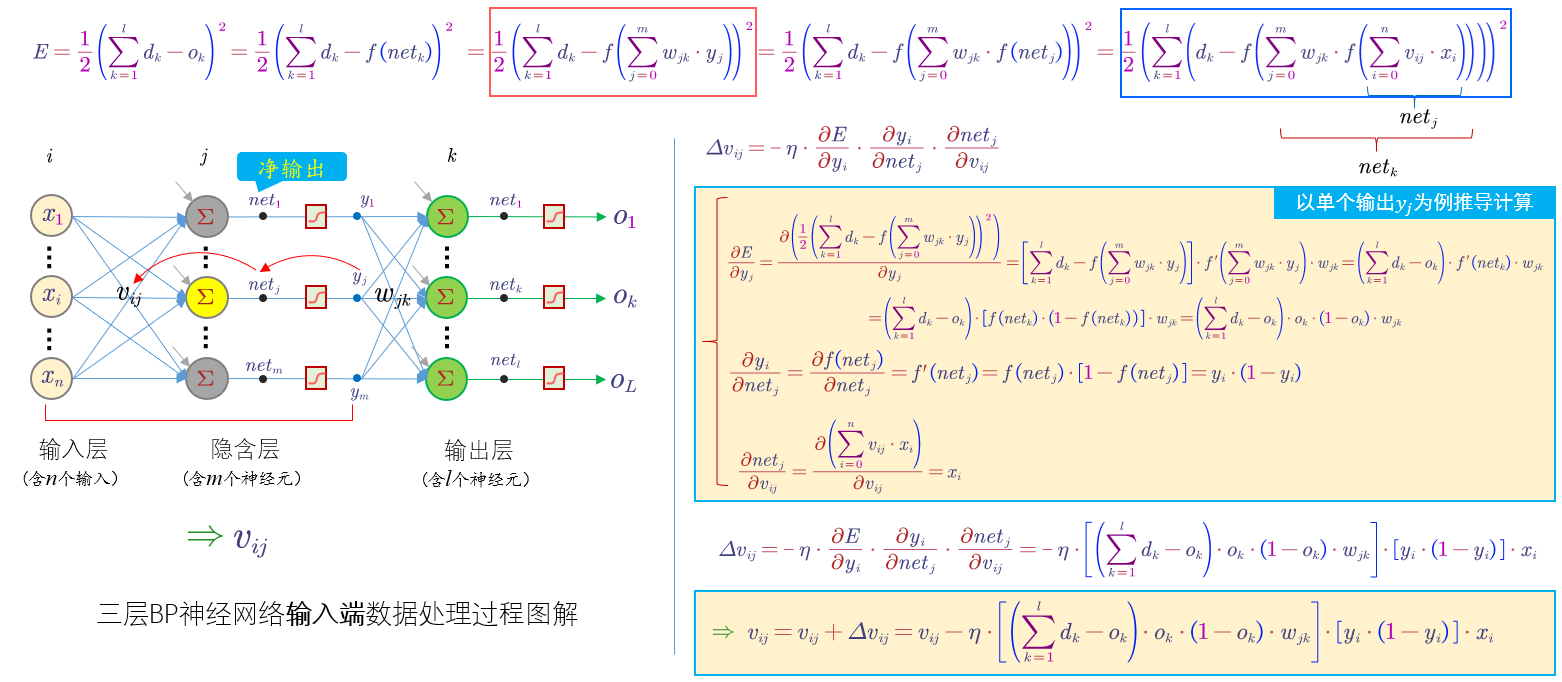欢迎转载，转载时请注明出处，谢谢！

展开全文神经网络 算法 深度学习 python 机器学习
•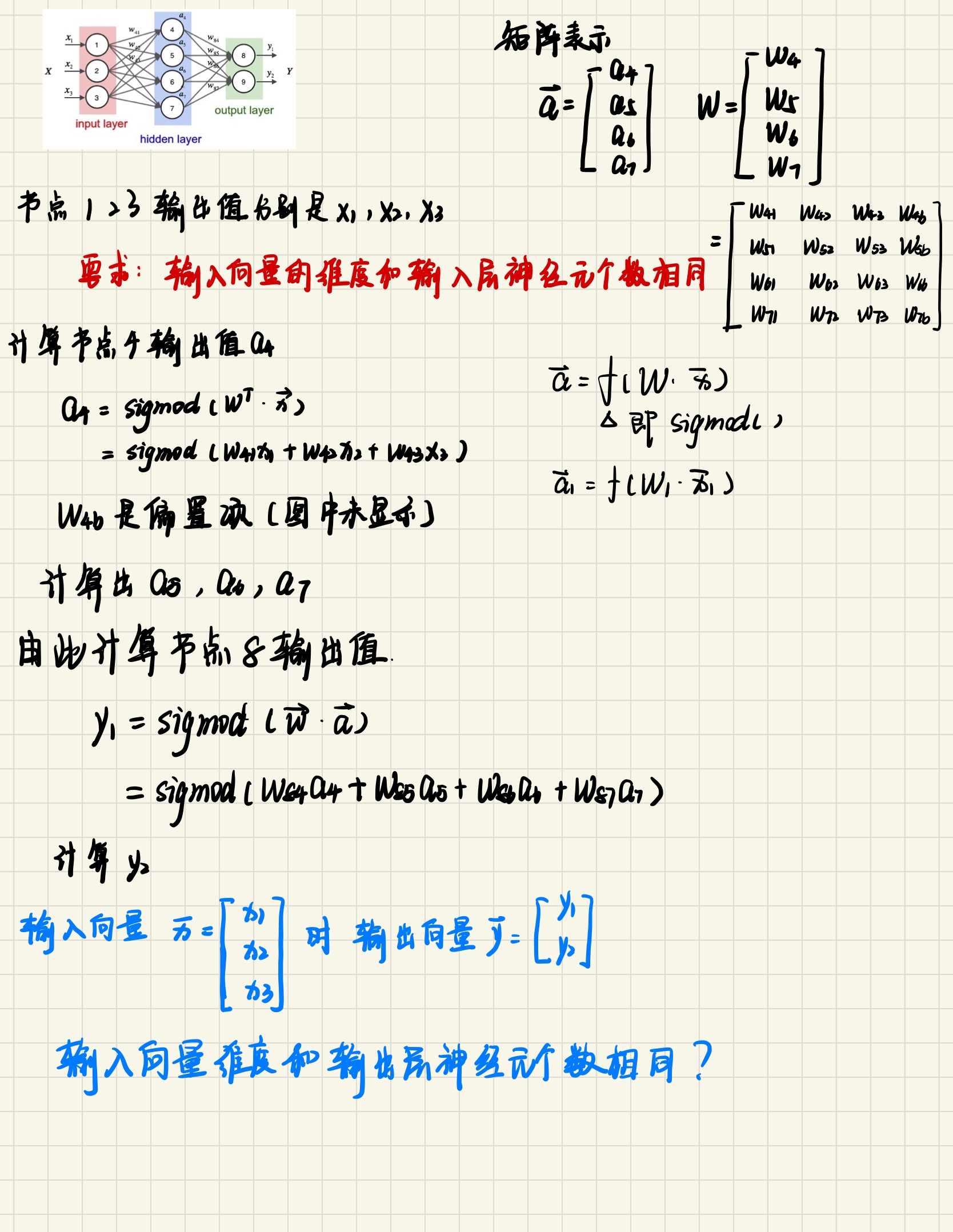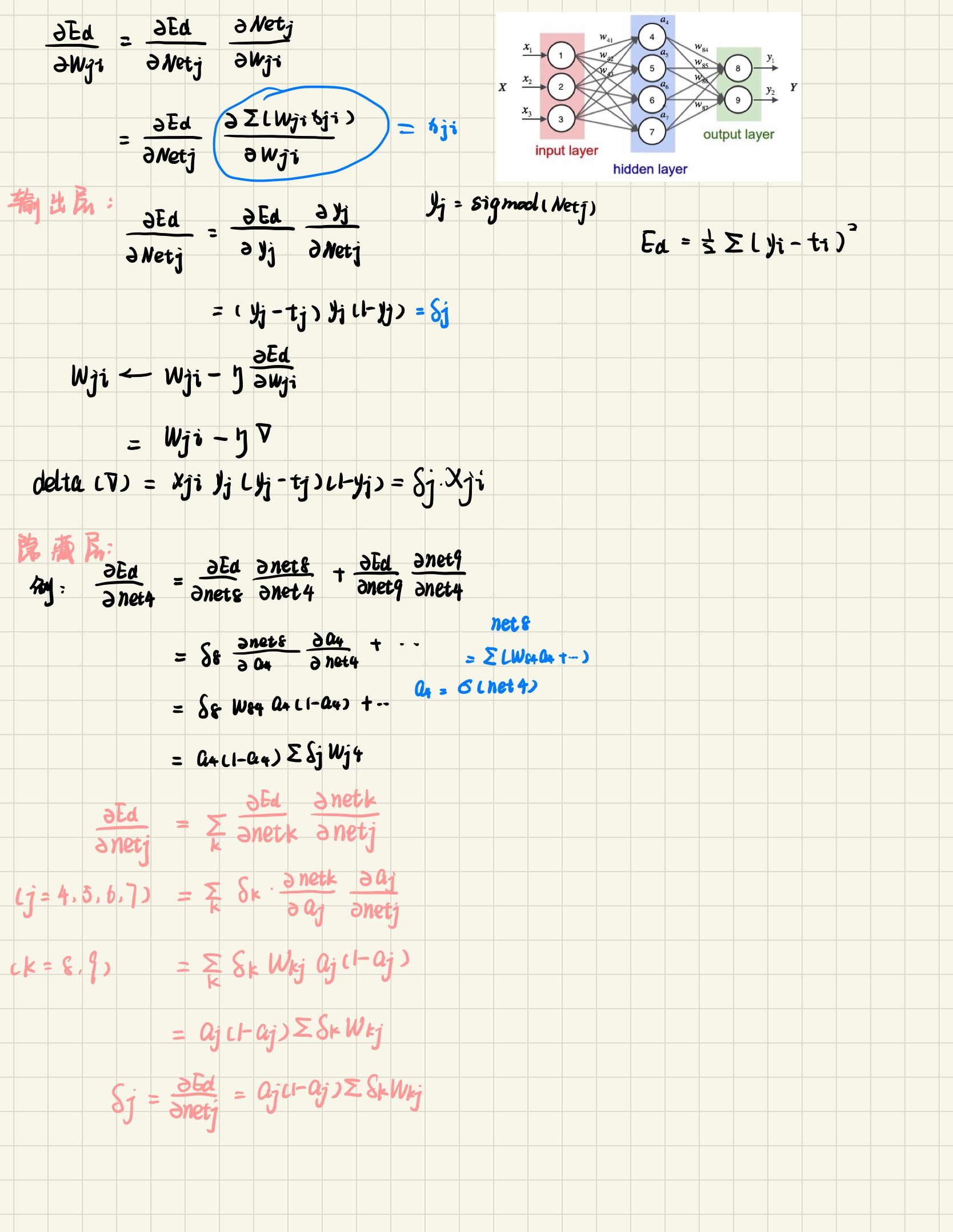展开全文•   写这个文章主要是因为学习了吴恩达老师的机器学习视频，在神经网络的反向传播算法（Back Propagation）的公式推导有些模糊，所以自己也查阅了很多资料，才基本搞明白其中的原理。所以希望通过写一下文章来进行...

1.前言

写这个文章主要是因为学习了吴恩达老师的机器学习视频，在神经网络的反向传播算法（Back Propagation）的公式推导有些模糊，所以自己也查阅了很多资料，才基本搞明白其中的原理。所以希望通过写一下文章来进行梳理一下。
因为本文的公式推导是基于吴恩达老师的课程，课程里的神经网络部分主要讲述的是神经网络在分类的应用，因此激活函数采用的是sigmoid函数，下面的公式推导也基于这个进行推导。
最后，因为自己是刚开始学习机器学习，所以可能理解上存在一些小的偏差。如果文章中有错误，欢迎大家指正。

2.前向传播

要理解反向传播，那么就必须先理解前向传播，下图以一个三层神经网络为例。。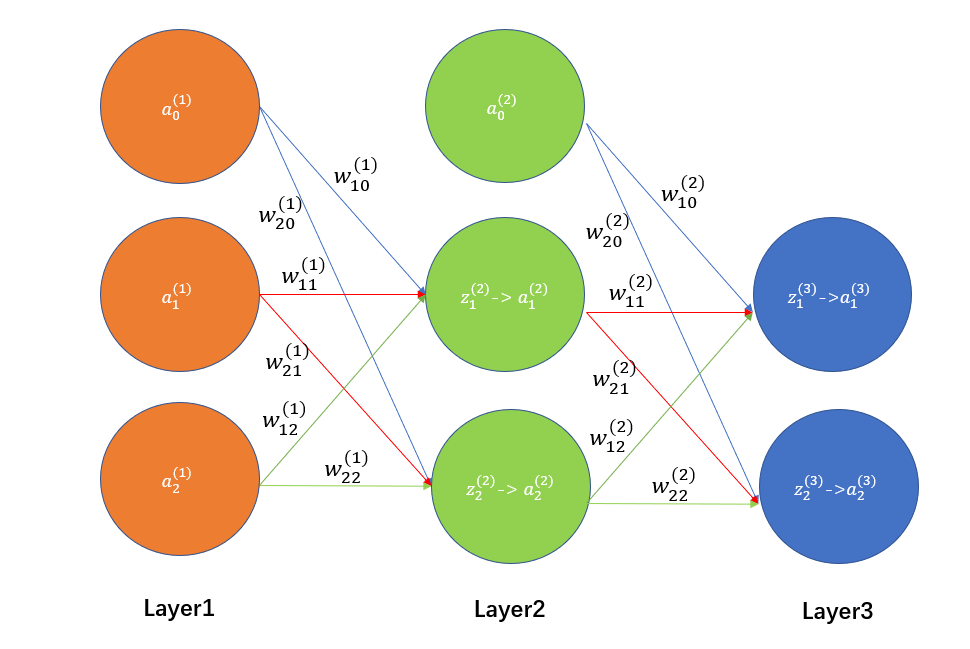根据上图，我给大家罗列一下正向传播的步骤，前向传播还是比较好理解的。通过输入层(Layer1)将数据输入（注意要加上偏执单元a0(l)=1），然后经过隐藏层（Layer2）加工处理，最后通过输出层（Layer3）进行输出结果。

1、输入层输入训练集
输入层其实就是对应上图中的Layer1
我们会有训练集**(x,y)**，训练集中会包含许多的 (x(i),y(i))样本，每一个x(i)中又会包含许多的特征 xj(i)，如x1(i)、x2(i)等等。
然后我们将x(i)中的xj(i)输入到神经网络中，就成为了输入层中的 aj(0)，其中x1(i)=a1(1)、x2(i)=a2(1)
最后，为了计算方便，我们需要加上 偏置单元a0(1)=1

2、隐藏层计算zj(2)
第二步就是通过权重矩阵计算出zj(2)，具体的计算方法如下图所示：因为权重w和参数θ代表的是一个意思，因此对于上图可以进行向量化表示，具体如下图所示(@代表矩阵的方式相乘)：3、通过激活函数计算出aj(2)
通过上一步计算的zj(2)，经过激活函数sigmoid，计算出aj(2)，具体如下图所示4、计算输出层的aj(3)
原理和上面计算隐藏层的类似，这里就不再重复了，相类比就可以计算出aj(3)

3.反向传播

还是老规矩，我们先上图，再一步一步进行解释。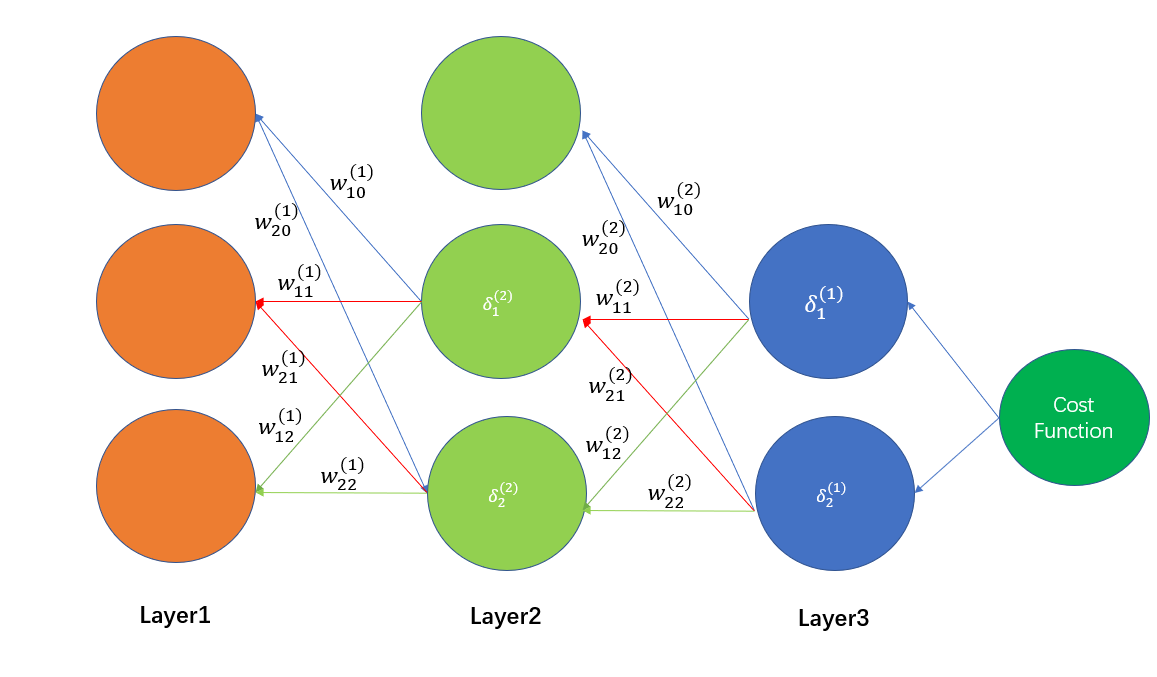反向传播的提出其实是为了解决偏导数计算量大的问题，利用反向传播算法可以快速计算任意一个偏导数。反向传播算法的思想和前向传播是一样的，只是一个反向的过程，推导过程中注意链式法则，一层扣一环即可求得结果。
大家在看下面的推导的时候，一定记得高数中的链式法则，逐步推导就可以了，其实是一个很简单的过程。

1、定义损失函数
首先，我们先定义这个神经网络的损失函数，如下图所示2、定义误差δ
吴恩达老师在视频中将δ翻译为误差，其实这是一种广义上的误差。因为除了输出层之外，我们是无法直接得到每一层的误差的。
广义上定义这个误差是这样的，如下图所示：因为偏导数其实就是表明了自变量对因变量产生了多大的影响。因此对于上面这个图中关于δ的定义，我们也就可以理解为zj(l)这个数对最终输出层的计算的误差J(θ)产生了多大的影响，也就是这个数对于这个误差做出了多大贡献。最理想的状态是δ=0，也就是偏导数等于0，没有对误差最终的误差产生影响。然后我们就将把这个“贡献”称为误差。
后面的公式计算每一层的δj(l)其实都是基于这个定义进行推导的。至于为什么在输出层没有使用这个定义去求误差，而是直接使用δj(L)=aj(L)-y得到输出层的误差，大家可以看完全文后回头去看一下这篇文章：反向传播输出层误差 δ。这篇文章清晰地进行了证明，这是因为逻辑回归的损失函数凑巧的一个性质造成的，如果是其他的损失函数则需要按照定义去

3、第L层δ（输出层）的具体推导
首先，我们先定义误差δ的一般形式，如下图所示：对于输出层的误差推导如下图所示：4、第l层误差δ的具体推导
下面我们对上一步进行推广，得到普遍的l层的误差误差δ对上图结果向量化可得：上面的推导看似很复杂，其实就是遵循一个链式法则，我已经基本没有省略步骤了，应该是很详细了。推导的时候需要记得前向传播中a(l)是如何通过权重矩阵编程z(l+1)的，然后z(l+1)通过激活函数又会变成a(l+1)，只要遵循这个就可以一步一步推导得出结果。

5、计算得出θij(l)的偏导数
反向传播算法的最终目的就是为了方便计算偏导数，到这一步就可以计算偏导数了。下面先重新放上吴恩达老师的视频截图，如下图所示从上图中我们可以看到，吴恩达老师还把g’(a)展开成了g(a)*(1-g(a))的形式，这个其实是因为sigmoid函数的性质造成的，具体推导如下：关于偏导数的推导如下图所示:通过上面的推导，就可以完全解释吴恩达老师所有公式的原理了。

4.参考文章

展开全文神经网络 反向传播 吴恩达 机器学习 算法
• 此文章仅为本人的学习笔记，侵权删...1.反向传播算法（Backpropagation） 作用：调参 w ，求权重。 先看一下正向传播（forwardpropagation），它是由x求出y^\hat yy^​,再根据（y^,y\hat y,yy^​,y）计算出loss。 ...

此文章仅为本人的学习笔记，侵权删。

1. “反向传播算法”过程及公式推导（超直观好懂的Backpropagation）
这讲了正向传播和反向传播的具体过程。

2. 反向传播算法（过程及公式推导）
这篇文章讲了反向传播算法的推导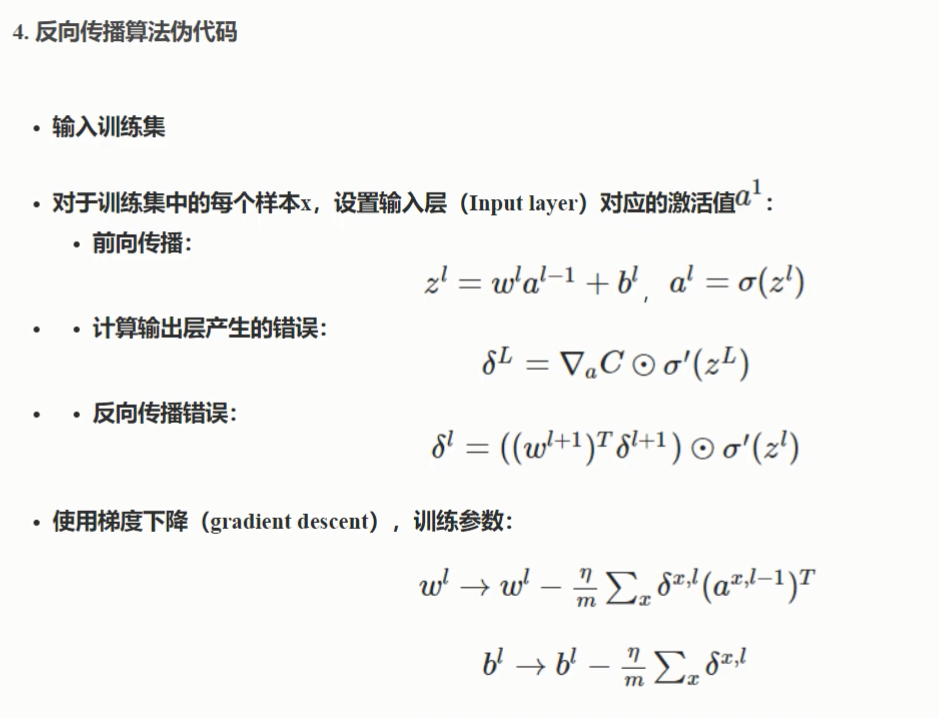代码练习：

数据集：数据

Python实现神经网络完成手写数字识别任务

激活函数是relu，输出层是softmax分类

import numpy as np
from sklearn.datasets import fetch_mldata
from sklearn.utils.extmath import safe_sparse_dot

# 由于输入层需要10个节点，所以最好把目标数字0-9做成One Hot编码的形式
def tran_y(y_true):
y_ohe = np.zeros(10)
y_ohe[int(y_true)] = 1
return y_ohe

mnist = fetch_mldata('MNIST original', data_home='data/for_my_own_nn_data/')

X, y = mnist["data"], mnist["target"]
print(X.shape)
print(y.shape)
y = np.array([tran_y(y[i]) for i in range(len(y))])

hidden_layer_sizes = [300, 100] #隐藏层个数：一个300，一个100
max_iter = 200
alpha = 0.0001  # 正则项系数
learning_rate = 0.001

(70000, 784)
(70000,)

def log_loss(y_true, y_prob): #交叉熵
"""
计算logistic loss对于分类任务
"""
y_prob = np.clip(y_prob, 1e-10, 1 - 1e-10)
if y_prob.shape == 1:
y_prob = np.append(1 - y_prob, y_prob, axis=1)
if y_true.shape == 1:
y_true = np.append(1 - y_true, y_true, axis=1)
return -np.sum(y_true * np.log(y_prob)) / y_prob.shape

def softmax(x): #实现softmax非线性变换
tmp = x - x.max(axis=1)[:, np.newaxis]
np.exp(tmp, out=x)
x /= x.sum(axis=1)[:, np.newaxis]
return x

def relu(x):
np.clip(x, 0, np.finfo(x.dtype).max, out=x)
return x

def relu_derivative(z, delta):
"""
实现relu的导数
"""
delta[z == 0] = 0#z==0执行，不等于0不执行

def gen_batches(n, bs):
"""
产生一个批次的样本数据的索引
:param n: 样本总数
:param bs: batch_size批大小
:return: 一个批次样本的索引
"""
start = 0
for _ in range(int(n // bs)):
end = start + bs
yield slice(start, end)
start = end
if start < n:
yield slice(start, n)

n_samples, n_features = X.shape
n_outputs = y.shape

batch_size = min(200, n_samples)
layer_units = ([n_features] + hidden_layer_sizes + [n_outputs])
#神经元个数：输入层神经元：[n_features] 隐藏：hidden_layer_sizes 输出层：[n_outputs]
n_layers = len(layer_units)#共有多少层

# 初始化W和b
coefs_ = []
intercepts_ = []
for i in range(n_layers - 1):#初始化各层的w与b
fan_in = layer_units[i]
fan_out = layer_units[i + 1]
# 推荐的初始化方法Xavier Glorot.论文得出
factor = 6.
init_bound = np.sqrt(factor / (fan_in + fan_out))
coef_init = np.random.uniform(-init_bound, init_bound, (fan_in, fan_out))
intercept_init = np.random.uniform(-init_bound, init_bound, fan_out)
coefs_.append(coef_init)
intercepts_.append(intercept_init)

# 初始化一些集合用于存放正向传播层层结果，反向传播层层梯度结果和中间数据deltas
# activations存放每一层的输出，输入层就是X，其它层先构建好输出的形状
activations = [X]
activations.extend(np.empty((batch_size, n_fan_out)) for n_fan_out in layer_units[1:]) #每层设置一个空矩阵来存储结果
deltas = [np.empty_like(a_layer) for a_layer in activations]#每层设置一个空矩阵来存储结果
coef_grads = [np.empty((n_fan_in_, n_fan_out_)) for n_fan_in_, n_fan_out_
in zip(layer_units[:-1], layer_units[1:])]
intercept_grads = [np.empty(n_fan_out_) for n_fan_out_ in
layer_units[1:]]

loss_ = 0.0

# mini batch 梯度下降
for it in range(max_iter):#轮次
arr = np.arange(n_samples)
np.random.shuffle(arr)
X = X[arr]
y = y[arr]
accumulated_loss = 0.0

for batch_slice in gen_batches(n_samples, batch_size):#批次
batch_X = X[batch_slice]
batch_y = y[batch_slice]
# 赋值输入层数据
activations = batch_X

# 正向传播
for i in range(n_layers - 1):
activations[i + 1] = safe_sparse_dot(activations[i], coefs_[i])
activations[i + 1] += intercepts_[i]
# 对于隐藏层
if (i + 1) != (n_layers - 1):
activations[i + 1] = relu(activations[i + 1])
# 对于最后一层输出层
activations[i + 1] = softmax(activations[i + 1])

# 计算平均Loss
loss = log_loss(batch_y, activations[-1])
# 给Loss添加L2正则项
values = np.sum(np.array([np.dot(s.ravel(), s.ravel()) for s in coefs_]))
loss += (0.5 * alpha) * values / len(batch_y)
accumulated_loss += loss * len(batch_y)

# 反向传播

# last是指反向传播从后面第一个要开始计的算层的索引号
last = n_layers - 2
# 这里计算delta[last]适用于输出非线性变换和损失函数的组合：
# softmax and categorical cross entropy
# 为了去计算倒数第一个W矩阵的梯度，先计算last对应的deltas
deltas[last] = activations[-1] - batch_y

# 计算倒数第一个W矩阵的梯度，即从输出层返回过来的梯度
# 1,base loss对应的梯度
# 2,L2 loss对应的梯度
# 3,梯度求平均
# 4,截距项,base loss对应的梯度

# 迭代计算各个隐藏层前面的W矩阵对应的梯度
for i in range(n_layers - 2, 0, -1):
# deltas_previous = deltas * W * 激活函数的导
deltas[i - 1] = safe_sparse_dot(deltas[i], coefs_[i].T)
# 应用上激活函数relu的导
relu_derivative(activations[i], deltas[i - 1])

# 计算每个隐藏层前面的W矩阵的梯度
# 1,base loss对应的梯度
coef_grads[i - 1] = safe_sparse_dot(activations[i - 1].T, deltas[i - 1])
# 2,L2 loss对应的梯度
coef_grads[i - 1] += (alpha * coefs_[i - 1])
# 3,梯度求平均
# 4,截距项,base loss对应的梯度
intercept_grads[i - 1] = np.mean(deltas[i - 1], 0)

# 梯度下降更新参数

# 这里的+号不是numpy数组之间的计算，而是python原生的list列表拼接
# grads: list, length = len(coefs_) + len(intercepts_)
# Wt+1 = Wt - learning_rate * grad
# params: list, length = len(coefs_) + len(intercepts_)
params = coefs_ + intercepts_
for param, update in zip(params, updates):
param += update

loss_ = accumulated_loss / X.shape
print("Iteration %d, loss = %.8f" % (it, loss_))

# TO DO ：
# 连续10次loss变换幅度小于阈值, break跳出

# TO DO ：
# 一次迭代后调整一次学习率

# TO DO ：
# train_test_split评估一下测试集准确率

Iteration 0, loss = 16.41843381
Iteration 1, loss = 12.05028002
Iteration 2, loss = 9.59518521
Iteration 3, loss = 8.07381126
Iteration 4, loss = 7.05051051
Iteration 5, loss = 6.34085800
Iteration 6, loss = 5.80947574
Iteration 7, loss = 5.39779761
Iteration 8, loss = 5.06627548
Iteration 9, loss = 4.78721871
Iteration 10, loss = 4.55101589
Iteration 11, loss = 4.35204685
Iteration 12, loss = 4.17534207
Iteration 13, loss = 4.02260116
Iteration 14, loss = 3.88357171
Iteration 15, loss = 3.76303320
Iteration 16, loss = 3.65209822
Iteration 17, loss = 3.55253729
Iteration 18, loss = 3.46278989
Iteration 19, loss = 3.37840236
Iteration 20, loss = 3.30269155
Iteration 21, loss = 3.23202948
Iteration 22, loss = 3.16538411
Iteration 23, loss = 3.10627120
Iteration 24, loss = 3.04794362
Iteration 25, loss = 2.99438211
Iteration 26, loss = 2.94299062
Iteration 27, loss = 2.89611537
Iteration 28, loss = 2.85032957
Iteration 29, loss = 2.80667936
Iteration 30, loss = 2.76552825
Iteration 31, loss = 2.72595690
Iteration 32, loss = 2.68790869
Iteration 33, loss = 2.65275958
Iteration 34, loss = 2.61760695
Iteration 35, loss = 2.58602208
Iteration 36, loss = 2.55289127
Iteration 37, loss = 2.52326688
Iteration 38, loss = 2.49402990

展开全文算法 深度学习 机器学习 神经网络 人工智能
• 1.如果使用矩阵对矩阵求导，还需要学习许多新知识，使原本简单的计算变得曲折，即使求出导数，但得到的导数与反向传播的输入是选择左乘还是右乘，作者没有说明，这就导致这个方法陷入停滞。 2.链式法则是针对标量的...深度学习
• Softmax 与交叉熵损失函数的反向传播公式推导 一、正向传播 用一个 X 举例，假设 S 为最后一层全连接层的输出，S 是一个长度为 c 的行向量，其中元素的含义为 c 个类分别的得分，即 s1s_1s1​ 为 X 在第一个类的得分...神经网络 深度学习 机器学习 反向传播
• 作者丨南柯一梦宁沉沦@知乎（已授权）来源丨https://zhuanlan.zhihu.com/p/61898234编辑丨极市平台导读在本篇文章中我们将从直观感受和数学公式两方面来介绍C...卷积 神经网络 算法 计算机视觉 机器学习
• 误差反向传播算法是通过误差函数计算实际输出值与期望输出值之间的误差，把误差从最后的输出层依次传播到之前各层，最后通过调整各层连接权重与偏置达到减小误差的目的。而权重和偏置的调整一般使用梯度下降法。 ...深度学习 神经网络 算法 梯度下降法 误差反向传播
• 文章目录九、神经网络参数的反向传播算法9.1 代价函数9.2 反向传播算法9.3 反向传播算法的直观理解9.5 梯度检验9.6 随机初始化9.7 总结 九、神经网络参数的反向传播算法 9.1 代价函数 首先引入一些新标记方法： ...神经网络 机器学习 算法 反向传播
• 现在才意识到，卷积神经网络在不同的层上的反向传播计算公式不一样，之前一直按照全连接层的那种简单反向传播去理解了。 全连接层反向传播 在数据表示上，将全连接神经网络的每一层神经元都表示为一个列向量。每...神经网络 深度学习 机器学习
• 从前面的学习中，我们学习到多层输出感知机的梯度计算公式为 在这里，我们通过代替作为一种方式，即此时原公式变为 其中中包含k个变量，即...。 对于再多一层的模型，反向求梯度即为 其中即为经过线性激活函数...pytorch 算法 人工智能
• 数学公式推导_MLP反向传播算法_Multi_Layer Perceptron 简化公式 ∂E∂wjk=(Ok−tk)Ok(1−Ok)OJj \frac{\partial E}{\partial w_{jk}}=\left( O_k-t_k \right) O_k\left( 1-O_k \right) O_{J}^{j} ∂wjk​∂E​=(Ok...pytorch
• 首先，写出整体的偏导计算公式，如下： ∂ C ∂ w 11 F 1 = ∂ C ∂ z 11 F 1 ∂ z 11 F 1 ∂ w 11 F 1 + ∂ C ∂ z 12 F 1 ∂ z 12 F 1 ∂ w 11 F 1 + . . . + ∂ C ∂ z 44 F 1 ∂ z 44 F 1 ∂ w 11 F 1 = ∂ C ...神经网络 算法 深度学习 误差反向传播算法
• 点击上方“小白学视觉”，选择加"星标"或“置顶”重磅干货，第一时间送达 本文转自：机器学习算法那些事阅读本文之前，可以先阅读之前讲述的全连接层的反向传播算法详细推导过程，...卷积 人工智能 计算机视觉 图像识别 opencv
• 最近看了两篇关于FPGA做训练的文章，发现在FPGA做训练很少有人做，就觉得肯定是哪里有问题导致大家不愿意做训练，于是研究了一下CNN的反向传播。但是发现网上的blog大多只是简单的从理论角度推导了一下最基本的情况...机器学习 卷积神经网络 python 算法
• 可惜单个神经元效果并不好，好吧，利用单个神经元做分类也不是我们的目的，但是学好神经元对于构建神经网络意义重大，我希望你能认真查看神经元的构建和推导的公式。本文也将进行神经网络的推导，相关知识，包括链式...神经网络 网络 深度学习 人工智能 机器学习
• 一：DNN的反向传播算法 我们在监督学习中的一般问题是，假设我们有m个训练样本，{(x1,y1),(x2,y2),…,(xm,ym)}，其中x是输入维度，输入特征维度为n_in，y为输出向量，输出的特征唯独为n_out,.我们需要利用这m个训练...算法 dnn 机器学习
• （3）多变量函数的梯度下降示例 我们发现，已经基本靠近函数的最小值点 5、梯度下降法的优化 （1）随机梯度下降法 （2）动量法 三、反向传播算法 反向传播算法本质上是梯度下降法。 梯度下降可以应对带有明确求导...神经网络 机器学习
• 03—BP算法推导3.1 公式1如下图所示，有个精灵跑到了网络中，假设位于第L层，第 j 个神经元的门口处，它引起了一点扰动，， z的含义是加权输入项，容易得出这个扰动项对成本函数造成的损失可以定义为： 那么，类推的...cnn 反向传播推导
• 2 反向传播算法原理 反向传播算法就类似于我们高数中所学的复合函数求导，例如两层的神经网络，就类似于两层的复合函数 ，这时求 对x的导数，就可以先计算，再计算，两数相乘就计算出了。如图所示是反向传播算法...深度学习
• 本文的反向传播算法的推导过程根据的是交叉熵代价函数，并非二次代价函数。不同代价函数的求导结果不同所以结果略有差异，但本质都是相同的。 交叉熵代价函数: J(Θ)=−1m∑i=1m(y(i)log(hθ(x(i)))+(1−y(i))log(1...人工智能
• 反向传播是神经网络通过调整神经元的权重和偏差来最小化其预测输出误差的过程。但是这些变化是如何发生的呢?如何计算隐藏层中的误差?微积分和这些有什么关系?在本文中，你将得到所有问题的回答。让我们开始吧。 在...深度学习 神经网络 反向传播 机器学习
• 经典的反向传播已得到扩展和修改，产生了许多不同的训练算法。本章中将讨论神经网络最常用的训练算法。我们从经典的反向传播开始，然后以随机梯度下降结束本章。 6.1　理解梯度 反向传播是梯度下降的一种，许多教...反向传播训练 梯度下降 算法 人工智能 神经网络
• 反向传播算法代码实现 代码实现 import numpy as np import math import tensorflow as tf import matplotlib.pyplot as plt def grad_sigmoid(n): # 求sigmoid梯度 return np.array(tf.sigmoid(1.0 - tf....神经网络
• def f(x): # 计算输出值 y = x ** 2 * t.exp(x) return y def gradf(x): # 手动计算梯度 dx = 2 * x * t.exp(x) + x ** 2 * t.exp(x) return dx x = V(t.randn(3, 4), requires_grad=True) y = f(x
• 在反向传播时对输入数据计算导数值f’(x)，然后乘以后一层送入的误差项，得到本层的误差项，送入前一层中： 反向传播算法推导 介绍 反向传播算法从多元复合函数求导的链式法则导出，递推的计算神经网络每一层参数的...
• 七、反向传播算法 内容参考来自https://github.com/dragen1860/Deep-Learning-with-TensorFlow-book开源书籍《TensorFlow2深度学习》，这只是我做的简单的学习笔记，方便以后复习。 1.激活函数导数 1.1Sigmoid ...
•   求解计算图法中反向传播的目的在于计算结果的偏差对于前面各项参数的影响大小，应用于BP算法中对神经网络中的权重进行调整。当计算结果与真实结果不符，通过反向传播计算，可指导如何调节权重参数，以期结果...神经网络 机器学习
• 1、正向传播 LSTM的正向传播公式： ...由于传播过程比较复杂，我们画一个计算草图来反映时间步t的正向传播中，各个量的关系： ...计算图中红色部分不属于时间...这对于理解反向传播中、的导数从何而来非常重要，在...神经网络 lstm...